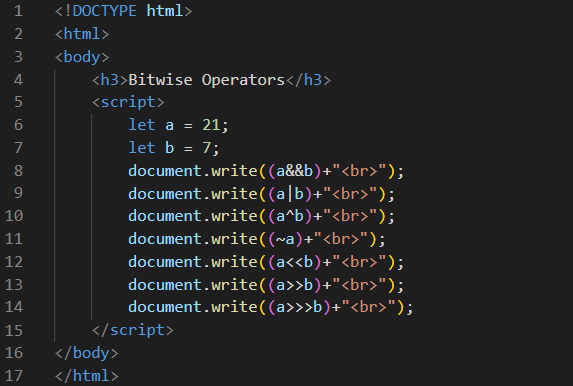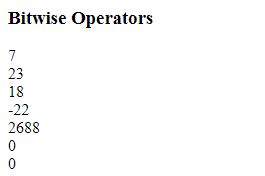JavaScript Bitwise Operators | Javascript Bitwise Tutorial By WDH

# JavaScript Bitwise Operators

JavaScript Bitwise Operators
All numbers stored as 64 bits floating point in JavaScript.
All operations are performed on 32 bits binary numbers.
Therefore, 64 bits floating point numbers are converted to 32 bits integers by JavaScript before performing bitwise operation.
There result of bitwise operation is a signed 32-bit integer
The result of the bitwise operation is converted back to a 64 bits JavaScript number.
The bitwise Operators are as shown below:
 Operator Name Description & AND Sets each bit to 1 if both bits are 1 | OR Sets each bit to 1 if one of two bits is 1 ^ XOR Sets each bit to 1 if only one of two bits is 1 ~ NOT Inverts all the bits << Zero fill left shift Shifts left by pushing zeros in from the right and let the leftmost bits fall off >> Signed right shift Shifts right by pushing copies of the leftmost bit in from the left, and let the rightmost bits fall off >>> Zero fill right shift Shifts right by pushing zeros in from the left, and let the rightmost bits fall off

ExampleOutput